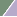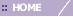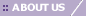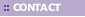# Euclidean and Euclidean Squared

Overview

Euclidean Distance Metric:

The Euclidean distance function measures the ‘as-the-crow-flies’ distance. The formula for this distance between a point X (X1, X2, etc.) and a point Y (Y1, Y2, etc.) is: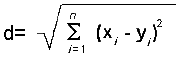Deriving the Euclidean distance between two data points involves computing the square root of the sum of the squares of the differences between corresponding values.

The following figure illustrates the difference between Manhattan distance and Euclidean distance: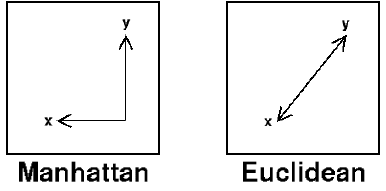Euclidean Squared Distance Metric

The Euclidean Squared distance metric uses the same equation as the Euclidean distance metric, but does not take the square root. As a result, clustering with the Euclidean Squared distance metric is faster than clustering with the regular Euclidean distance. The output of Jarvis-Patrick and K-Means clustering is not affected if Euclidean distance is replaced with Euclidean squared. However, the output of hierarchical clustering is likely to change.

Related Topics:

Clustering Overview